﻿ Variation of Excess Minority Carriers Density in Function to Silicon Solar Cell Paremeters Involving Excitons Effects

### Variation of Excess Minority Carriers Density in Function to Silicon Solar Cell Paremeters Involving...

Mamadou NIANE, Modou FAYE, Saliou NDIAYE, Modou PILOR, Ousmane NGOM, Moulaye DIAGNE, Nacire MBINGUE, Omar. A. NIASSE, Bassirou BA

Physics and Materials Chemistry

## Variation of Excess Minority Carriers Density in Function to Silicon Solar Cell Paremeters Involving Excitons Effects

Mamadou NIANE1,, Modou FAYE1, Saliou NDIAYE1, Modou PILOR1, Ousmane NGOM1, Moulaye DIAGNE1, Nacire MBINGUE1, Omar. A. NIASSE1, Bassirou BA1

1Laboratoire de Semi-conducteurs et d’Energie Solaire, Département de Physique, Faculté des Sciences et Techniques (UCAD-SENEGAL)

### Abstract

In this work, we have studied the variations of excess minority carriers density in the base in function to some parameters to the silicon solar cell taking into account excitons effects. To do this, we have taken the expressions of excess electrons density in the base obtained by resolution of the differential equations of charge transport in static condition, the study of here variations are done in function to some cell parameters such as the binding coefficient between electrons and excitons and the base thickness in strong coupling. The profile of the excess electron variation in function to the base thickness for a strong coupling shows that, at the base junction, he is independent to the binding coefficient. This invariance is done to the intervention of the electric field that prevails in the depletion region which dissociates almost all excitons arriving at the junction. In depth, a strong coupling coefficient decreases the excess minority carriers density due to recombination that occur with excitons. The excess electrons density variation enabled us to know that when the coupling is strong, the doping level decreases the electrons density caused by the increase of recombination region due to the introduction of many impurities.

• Mamadou NIANE, Modou FAYE, Saliou NDIAYE, Modou PILOR, Ousmane NGOM, Moulaye DIAGNE, Nacire MBINGUE, Omar. A. NIASSE, Bassirou BA. Variation of Excess Minority Carriers Density in Function to Silicon Solar Cell Paremeters Involving Excitons Effects. Physics and Materials Chemistry. Vol. 4, No. 1, 2016, pp 10-14. http://pubs.sciepub.com/pmc/4/1/3
• NIANE, Mamadou, et al. "Variation of Excess Minority Carriers Density in Function to Silicon Solar Cell Paremeters Involving Excitons Effects." Physics and Materials Chemistry 4.1 (2016): 10-14.
• NIANE, M. , FAYE, M. , NDIAYE, S. , PILOR, M. , NGOM, O. , DIAGNE, M. , MBINGUE, N. , NIASSE, O. A. , & BA, B. (2016). Variation of Excess Minority Carriers Density in Function to Silicon Solar Cell Paremeters Involving Excitons Effects. Physics and Materials Chemistry, 4(1), 10-14.
• NIANE, Mamadou, Modou FAYE, Saliou NDIAYE, Modou PILOR, Ousmane NGOM, Moulaye DIAGNE, Nacire MBINGUE, Omar. A. NIASSE, and Bassirou BA. "Variation of Excess Minority Carriers Density in Function to Silicon Solar Cell Paremeters Involving Excitons Effects." Physics and Materials Chemistry 4, no. 1 (2016): 10-14.

 Import into BibTeX Import into EndNote Import into RefMan Import into RefWorks

12
Prev Next

### 1. Introduction

The electron transport equations and holes in the silicon base were established according to some conditions by R. Corkish et al. . The excess minority carriers density expression in the base obtained after calculation enable us to study, starting from numerical simulations, their variations in function to the some cell parameters taking account of the excitons effects.

The study related, initially, the variation of the excess electron density in function to the base depth when it is considered that the coupling between electrons and excitons is strong. Then it related in the second party, the variation of the excess minority carriers density at the junction in function to the binding coefficient for different value of doping level values.

I. THEORY

The transport equations of excess minority carriers and excitons in the base are given by the following differential equations system: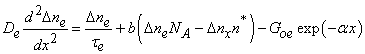(1.1)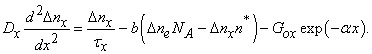(1.2)

The resolution of this system [1, 2] gives the following expressions of excess electrons density in the base:

In dark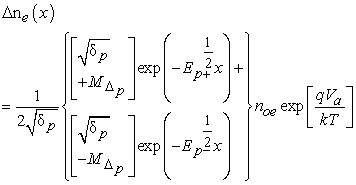(1.3)

In illumination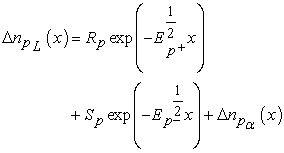(1.4)

With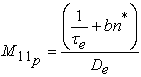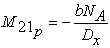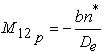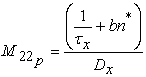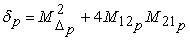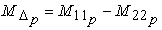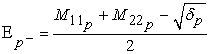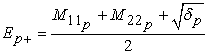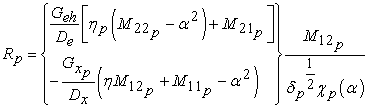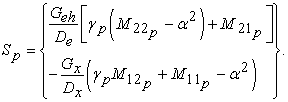(1.5)

These different expressions enable us to study the variations of the excess carriers in function to the cell parameters.

II. INFLUENCE OF EXCITONS ON THE EXCESS ELECTRONS DENSITY

The electron generation in the base is accompanied to a significant creation of excitons which interact with electrons [3, 4, 5, 6]. These interactions, when they are strong, caused a reduction of the mobility of the excess minority carriers density thus supporting an increase the recombination velocity in volume [7, 8, 9]. This study will make it possible to include well the variations of the electrons in the base in function to various cell parameters in strong coupling.

A. VARIATION OF EXCESS MINORITY CARRIES IN FUNCTION TO THE BASE THICKNESS

Many studies had to be done on the variation of the excess minority carriers density in dark and base illuminated [10, 11, 12]. In this work, we study the variation of the excess electron density in function to the base depth for different values of the binding coefficient between electrons and excitons. We obtain various profiles in dark and illumination.

In darkDownload asVeiw figureFigures index
Figure 1.a.

Variation of excess minority carriers density in function to the base thickness: ni=1.5.1010 cm-3, De=33 cm2.s-1, Dx=17 cm2.s-1, T=300K, τe=4.10-6 s, τx= 6.69.10-6 s, Va=0.5V, NA=1016 cm-3Download asVeiw figureFigures index
Figure 1.b.

Variation of excess minority carriers density in function to the base thickness: ni=1.5.1010 cm-3, De=33 cm2.s-1, Dx=17 cm2.s-1, T=300K τe=4.10-6 s, τx= 6.69.10-6 s, Va=0.5V, NA=1016 cm-3

The profiles obtained above show that the variation of the excess minority carriers density is function to the coupling level between electrons and excitons. It decreases as the coupling increases. This is indeed comprehensible because a strong coupling caused by a strong Coulomb attraction between electrons and excitons decreases the electron mobility and supports consequently an increase of recombination velocity in volume. The high value density obtained at the junction is due to the intervention of the electric field of the depletion space charge which supports the dissociation of excitons and consequently the electron formation [13, 17].

The profile obtained in illumination shows that the excess minority carriers density increased in illuminated cell. We notice that in illumination, the excess minority carriers density at the base junction increases to 1011 cm-3 at 1012 cm-3 or to 1013 cm-3 in function to the binding coefficient value. This packing of the excess minority carriers density is due to an increase of the carriers generation rate following photons absorption of the valence band electrons or intermediary band towards the conduction band. The minimal value of the density at back face is due to a very significant recombination rate in this region caused by the metal contact [18, 19, 20, 21].

B. VARIATION OF EXCESS MINORITY CARRIERS IN FUNCTION TO BINDING COEFFICIENT

The electrons and the excitons are bound by Coulomb interactions materialized by a binding coefficient noted b. This coefficient highlights the interaction level between electrons and holes, it varies from 10-7 to 10-15 cm3.s-1. Its value is function to the coupling nature, it is very weak (b≈10-15cm3.s-1) if excitons effect is neglected and it is said whereas the coupling is weak. On the other hand, b>10-9 cm3.s-1 when the excitons effects are very significant, the coupling is strong. These figures below give the excess minority carriers density variation in function to the binding coefficient.Download asVeiw figureFigures index
Figure 2.

Variation of excess minority carriers in function to binding coefficient ni=1.5.1010 cm-3, De=33 cm2.s-1, Dx=17 cm2.s-1, T=300K, τe=4.10-6 s, τx= 6.69.10-6 s, Va=0.5V, NA=1016 cm-3Download asVeiw figureFigures index
Figure 3.

Variation of excess minority carriers in function to binding coefficient ni=1.5.1010 cm-3, De=33 cm2.s-1, Dx=17 cm2.s-1, T=300K, τe=4.10-6 s, τx= 6.69.10-6 s, Va=0.5V, NA=1016 cm-3

The observation of these profiles shows that the excess minoritycarriers density at the junction is independent to the binding coefficient. This confirms well the profiles obtained in Figure 1.a. and Figure 1.b. because in these three profiles, the electrons density obtained following different value of the binding coefficient converges all to a same value at the junction. This invariance in function to the binding coefficient is very comprehensible because the electrons arriving at the junction are almost free and do not exert any electric interaction with the holes . The binding coefficient highlighting the electrons and excitons interactions level is infinitely weak at the junction.

For studding the variation of the excess electrons density in function to the binding coefficient, a study was made following various positions in the base depth. We obtain thus the Figure 4.Download asVeiw figureFigures index
Figure 4.

Variation of excess minority carriers in function to binding coefficient (x=0μm, x=4μm, x=10μm, x=Hμm) ni=1.5.1010 cm-3, De=33 cm2.s-1, Dx=17cm2.s-1, T=300K, τe=4.10-6 s, τx= 6.69.10-6 s, NA=1016 cm-3.

This profile shows an invariance of the electrons density at the junction and the back face. But we notice that, for different positions in the base depth, a decrease of the density in function to the binding coefficient. This decrease is explained that in depth, when the coupling is strong, we obtain many interactions between electrons and holes. These interactions put the electron in a potential well which strongly reduces its mobility and thus decreasing its diffusion length. This cause thus an increase of the electrons recombination velocity and reduces consequently the excess minority carriers density.

The profiles obtained at the junction and the back face show that in these regions, the electrons density is independent to the binding coefficient. In the back face, the metal contact having no perfect adherence and the significant disturbance created in the crystal lattice by the metal contact welding; will constitute a Shockley-Read and Hall recombination center. The excess minority carriers recombination velocity is very high in this region. In other words their lifetime is very weak, so that the electrons density is equal to the equilibrium density.

### 2. Conclusion

This permit us to understand the excitons influence on the excess minority carriers density variations in the base in function to various cell parameters. The binding coefficient which highlights Coulomb interactions level between electrons and holes is very important in this study. Its low value (weak coupling) indicates a weak connection of the electron, the excitons generation is very negligible in this case. On the other hand, its high value is showing a strong interaction between the electrons and holes (strong coupling) causing an increase of the excitons recombination velocity and consequently a reduction of the excess minority carriers density in the density in the base. It should be noted that for a strong coupling, a high doping level reduce excess carriers density caused by shielding phenomenon of which occurs between carriers.

### Nomenclature

symbols: Name and unit

∆n: Excess minority carriers density, cm-3

∆nx: Excess excitons density, cm-3

b: Binding coefficient, cm3.s-1

Geh0: direct generation rate of carrier pairs, cm-3.s-1

Gx0: Excitons generation rate at the semiconductor surface, cm-3.s-1

∆noe: Excess minority carriers density at the junction, cm-3

x: The base thickness, cm

NA: Doping level, cm-3

De: Diffusion coefficient for electron, cm2.s-1

Dx: Diffusion coefficient for excitons, cm2.s-1

  R. Corkish, Daniel S-p. Chan and M. A. Green, “excitons in Silicon Diodes and Solar Cells - A Three Particle Theory”, Journal of Applied Physics, vol. 79, pp. 195-203, 1996In article View Article  Mamadou Niane, Omar. A. Niasse, Moulaye Diagne, Nacire Mbengue, Bassirou Ba, “Laplace transform calculation of dark saturation current in silicon solar cell involving exciton effects”, INTERNATIONAL JOURNAL OF ENGINEERING SCIENCES & RESEARCH TECHNOLOGY, vol. 4(3), pp. 279-285, mars 2015.In article  M. BALKANSKI « état actuel du problème de l’exciton » JOURNAL DE PHYSIQUE ET LE RADIUM TOME 19, FÉVRIER 1958, page 170.In article  J. Barrau, M. Heckmann, M. Brousseau, Determination experimentale du coefficient de formation d'excitons dans le siliciumJournal of Physics and Chemistry of Solids, 34, 3, 1973, 381-385In article  R.S. Knox, Theory of Excitons, Academic Press, New York, 1963.In article  Arthur J. Nozik, Multiple exciton generation in semiconductor quantum dots, Chemical Physics Letters 457 (2008) 3-11.In article View Article  EADES W. D and SWANSON R.M, Calculation of surface generation and recombination velocities at the Si-SiO2 interface, Journal of Applied Physics, vol. 58, 1995, 4267 p.In article  I. Zerbo, F. I. Barro, B. Mbow, A. Diao, F. Zougmore, G. Sissokho “theoretical study of bifacial silicon solar cell under frequency modulated while light: Determination of recombination parameters. 19thEuropean Photovoltaique solar conference and exhibition June 2004, Paris France.In article  Sproul A. B., Dimensionless solution of the equation describing the effect of surface ecombination on carrier decay in semiconductors, J. Appl. Phys., vol.76, Issue 5, 1994, pp. 2851-2854.In article View Article  A. Green “Concentration and Minority-Carrier Mobility of Silicon from 77-300K”, Journal of Applied Physics, Vol. 73, pp. 1214-1225, 1993.In article View Article  A. Green and J. Shewchun, “Minority Carrier Effects Upon the Small Signal and Steady-State Properties of Schottky Diodes”, Solid-State Electronics, pp. 1141-1150, 1973.In article View Article  SHOCKLEY W, READ W.T.Jr. Statistics of the Recombinations of Holes and Electron. Physical. Review, vol. 87, 1952, pp. 835-842.In article View Article  Halls, Pichler, Friend, Moratti, Holmes« Exciton dissociation at a PPV/ C60 heterojunction » Synthetic Metals 77 (1996) 277-280.In article View Article  HALL R. N. Electron-Hole Recombination in Germanium, Physical Review, vol. 87 1952, 387 p.In article  Saliou NDIAYE, Mamadou Niane, Nacire Mbengue,Moulaye Diagne, Omar. A. Niasse, Bassirou Ba, “effects of temperature on the short circuit current of a silicon solar cell while taking into account the excitons”, INTERNATIONAL JOURNAL OF ENGINEERING SCIENCES & RESEARCH TECHNOLOGY, vol 4(3). pp. 441-444, mars 2015.In article  Luque A., Martı A., Eds., Next Generation PhotoVoltaics: High Efficiency through Full Spectrum Utilization; Institute of Physics, Bristol, UK, 2003.In article  Murphy, J. E.; Beard, M. C.; Norman, A. G.; Ahrenkiel, S. P.; Johnson, J. C.; Yu, P.; Mic´ic´, O. I.; Ellingson, R. J.; Nozik, A. J. J. Am. Chem. Soc. 2006, 128, 3241.In article View Article  PubMed  EADES W. D and SWANSON R.M, Calculation of surface generation and recombination velocities at the Si-SiO2 interface, Journal of Applied Physics, vol. 58, 1995, 4267 p.In article  A.G. Aberle, S.J. Robinson, A. Wang, J. Zhao, S.R. Wenham and M.A. Green, “High Efficiency Silicon Solar Cells: Fill Factor Limitations and Non-Ideal Diode Behaviour Due to Voltage-Dependent Rear Surface Recombination Velocity”, Progress in Photovoltaics, Vol. 1, No. 2, pp. 133-143, 1993.In article View Article  A.W. Stephens, A.G. Aberle and M.A. Green, “Surface Recombination Velocity Measurements at Silicon/Silicon Dioxide Interface by Microwave-Detected Photoconductance Decay”, J. Appl. Phys., Vol. 76, pp. 363-370, 1994.In article View Article  Manna, L.; Milliron, D. J.; Meisel, A.; Scher, E. C.; Alivisatos, A. P. Nature Mater. 2003, 2, 382.In article View Article  PubMed  Marc Dvorak, Su-Huai Wei, and Zhigang Wu1, “Origin of the Variation of Exciton Binding Energy in Semiconductors” PHYSICAL REVIEW LETTERS 110, 016402 (2013).In article View Article  PubMed